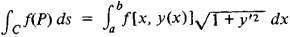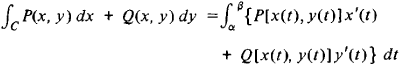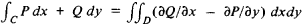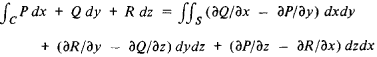# Line Integral

(redirected from Curve integral)

## line integral

[′līn ¦int·ə·grəl]
(mathematics)
For a curve in a vector space defined byx=x(t), and a vector functionVdefined on this curve, the line integral ofValong the curve is the integral over t of the scalar product ofV[x(t)] and d x/ dt ; this is written ∫V· d x.
For a curve which is defined by x = x (t), y = y (t), and a scalar function ƒ depending on x and y, the line integral of ƒ along the curve is the integral over t of ƒ[x (t), y (t)] · √(dx / dt)2+ (dy / dt)2); this is written ∫ ƒ ds, where ds = √(dx)2+ (dy)2) is an infinitesimal element of length along the curve.
For a curve in the complex plane defined by z = z (t), and a function ƒ depending on z, the line integral of ƒ along the curve is the integral over t of ƒ[z (t)] (dz / dt); this is written ∫ ƒ dz.
McGraw-Hill Dictionary of Scientific & Technical Terms, 6E, Copyright © 2003 by The McGraw-Hill Companies, Inc.
The following article is from The Great Soviet Encyclopedia (1979). It might be outdated or ideologically biased.

## Line Integral

an integral taken along some curve in the plane or in space. We distinguish line integrals of the first kind and line integrals of the second kind. A line integral of the first kind arises, for example, in problems involving the calculation of the mass of a curve of variable density and is denoted by

Cf (P)ds

where C is the given curve, ds is the differential of its arc, and f(P) is the function of a point on the curve and is the limit of the corresponding integral sums. In the case of a plane curve C given by the equation y = y(x), a line integral of the first kind reduces to an ordinary integral. Specifically,A line integral of the second kind arises, for example, in connection with the work of a force field. In the case of a plane curve C the integral takes the form

CP(x,y)dx + Q(x,y)dy

and is also the limit of the corresponding integral sums. A line integral of the second kind can be expressed as an ordinary integral. Specifically,wherex = x(t) and y = y(t) for α ≤ t ≤ β, is the parametric equation of the curve C. Its connection with a line integral of the first kind is given by the equality

CP(x,y)dx + Q (x,y)dy = ∫C {P cosα + Q sin α} ds

Here, α is the angle between the Ox axis and the tangent to the curve pointing in the direction of the increasing arc length.

A line integral of the second kind in space is defined similarly. (SeeVECTOR CALCULUS for a treatment of line integrals of the second kind from the standpoint of vectors.)

Suppose D is some region and C is its boundary. Under certain conditions, the line integral along the curve C and the double integral over the region D (see) are connected by the relationSimilarly, the line integral and the surface integral are connected by the relationLine integrals are of great importance in the theory of functions of a complex variable. They are widely used in various branches of mechanics, physics, and engineering.